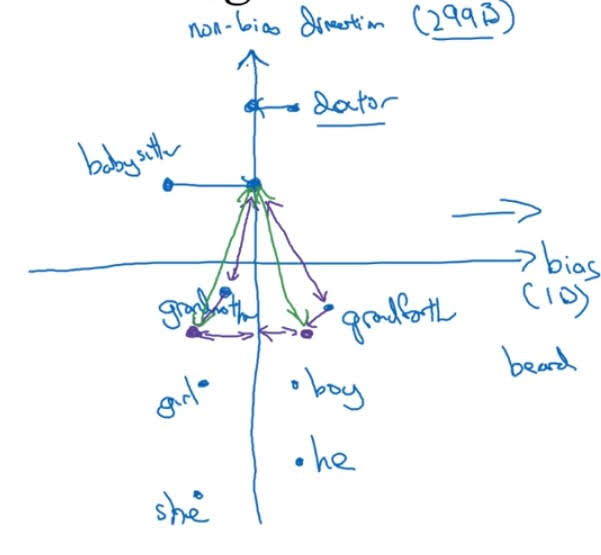# Debiasing Word Embeddings

Categories:

Updated:

https://arxiv.org/abs/1607.06520

We are building word embeddings from our previous usage of words. So word embeddings can reflect gender, ethnicity, age, sexual orientation, and other biases of the text used to train the model. For example, word embeddings can make analogies such as ‘man is to computer programmer as woman is to homemaker’.

Then how do we stop this? How do we debias word embeddings? I’ll talk about debiasing gender bias as an example.

### 1. Identify bias direction.

One way to identify bias direction is to average differences between opposite definitial words. For example, we average $e_{he}-e_{she}$ , $e_{male}-e_{female}$, ,…

Then the resulting vector is a ‘bias’ axis. In the below image, bias axis is drawn as x-axis and all the remaining dimentions are simplified into y-axis.### 2. Neutralize

For every word that is not definitial, project them to make bias 0. In the image above, we’ve projected ‘doctor’ and ‘babysitter’ to y-axis.

### 3. Equalize Pairs

Lastly, adjust definitial words so that they are of equal distance from non-bias axis. In the above image, we’ve adjusted ‘grandmother’ and ‘grandfather’ so that they have equal distance from non-bias axis.

You can see that ‘grandfather’ and ‘grandmother’ now have equal distance from ‘babysitter’.

Categories: